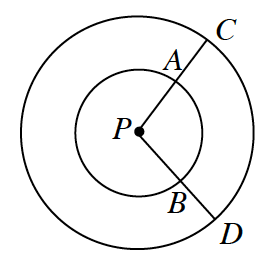### Home > GC > Chapter 10 > Lesson 10.1.3 > Problem10-29

10-29.The figure at right shows two concentric circles.

1. Which arc has greater measure: $\overarc{ A B }$ and $\overarc{ C D }$? Explain.

They are similar. Both are the intercepted arc of central angle $∠CPD$.

2. Which arc has greater length? Explain how you know.

We already know that both share the same central angle. Which one has a larger radius?

Both arcs have the same measure.

$\overarc{CD}$ has greater length.

3. If $m∠P = 60°$ and $PD=14$, find the length of $\overarc{ C D }$. Show all work.

Arc $\overarc{CD}$ is a fraction of a circle with a radius of $14$ and a circumference of $2πr = 2π(14) = 28π$.

Therefore, the length of $\overarc{CD} = \frac{60}{360} (28\pi)$.